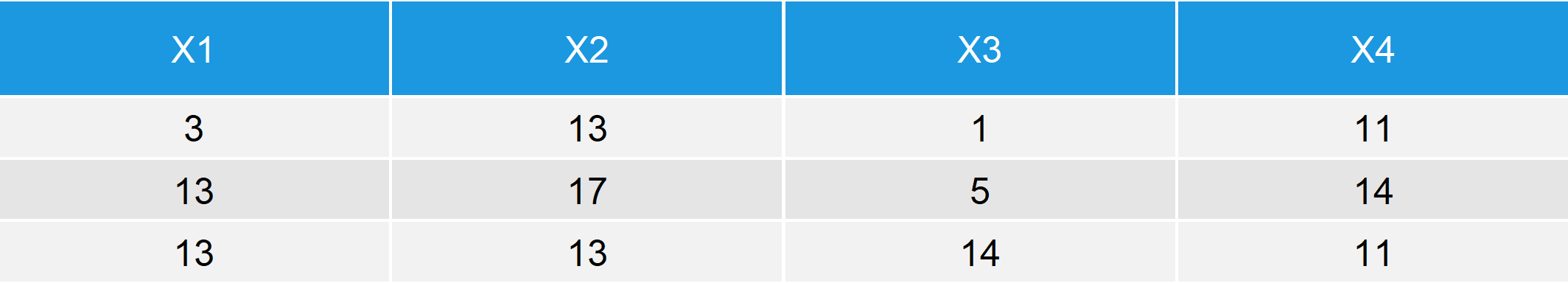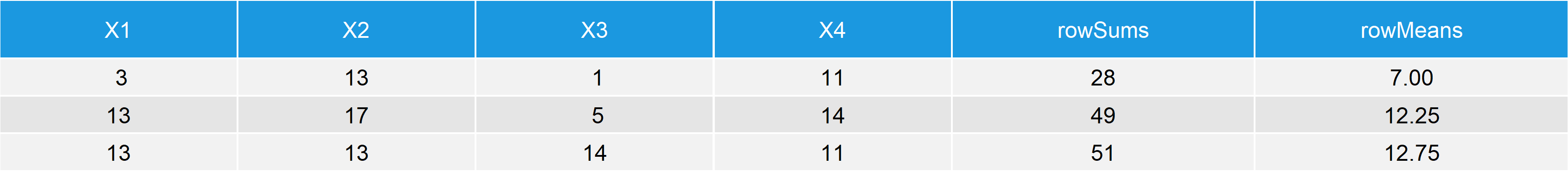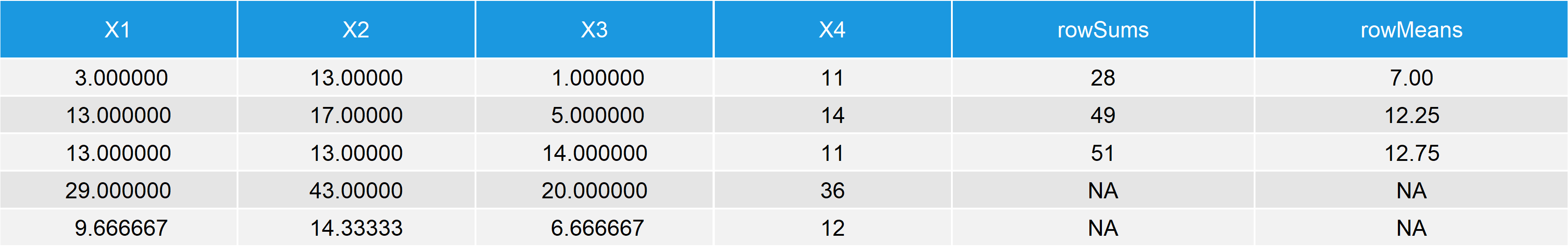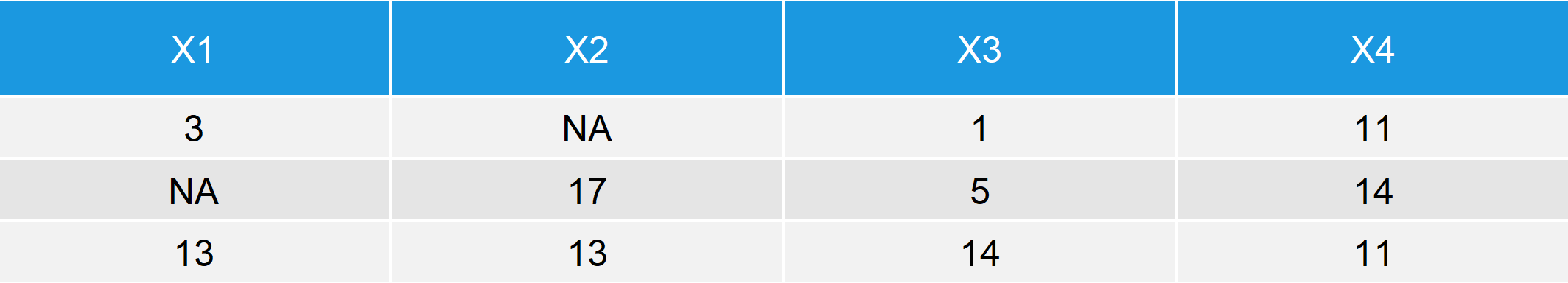# colSums, rowSums, colMeans & rowMeans in R | 5 Example Codes + Video

In this tutorial, I’ll show you how to use four of the most important R functions for descriptive statistics: colSums, rowSums, colMeans, and rowMeans.

I’ll explain all these functions within the same article, since their usage is very similar. Let’s first check the basic R programming syntax of the four functions:

Basic R Syntax:

```colSums(data) rowSums(data) colMeans(data) rowMeans(data)```

• colSums computes the sum of each column of a numeric data frame, matrix or array.
• rowSums computes the sum of each row of a numeric data frame, matrix or array.
• colMeans computes the mean of each column of a numeric data frame, matrix or array.
• rowMeans computes the mean of each row of a numeric data frame, matrix or array.

In the following, I’m going to show you five reproducible examples on how to apply colSums, rowSums, colMeans, and rowMeans in R.

So if you want to know more about the computation of column/row means/sums, keep reading…

## Example 1: Compute Sum & Mean of Columns & Rows in R

Let’s start with a very simple example. For the example, I’m going to use the following synthetic data set:

```set.seed(1234) # Set seed data <- data.frame(matrix(round(runif(12, 1, 20)), # Create example data nrow = 3, ncol = 4)) data # Print data to RStudio console```Table 1: Data Frame Containing Numeric Values.

Our example data consists of 3 rows and four columns. All values are numeric.

To this data set, we can now apply the four functions. Let’s compute the column sums

```colSums(data) # Basic application of colSums # X1 X2 X3 X4 # 29 43 20 36```

…the row sums…

```rowSums(data) # Basic application of rowSums # 28 49 51```

…the column means…

```colMeans(data) # Basic application of colMeans # X1 X2 X3 X4 # 9.666667 14.333333 6.666667 12.000000```

…and the row means:

```rowMeans(data) # Basic application of rowMeans # 7.00 12.25 12.75```

That’s basically how to apply the four functions! However, if you need more explanations you could have a look at the following video of my YouTube channel. In the video, I’m explaining Example 1 in more detail:

Please accept YouTube cookies to play this video. By accepting you will be accessing content from YouTube, a service provided by an external third party.If you accept this notice, your choice will be saved and the page will refresh.

## Example 2: Add Sums & Means to Data Frame

Typically, we would like to add the computed mean and sum values to our data frame. We can easily column-bind the rowSums and rowMeans with the following code:

```data_ext1 <- cbind(data, # Add rowSums & rowMeans to data rowSums = rowSums(data), rowMeans = rowMeans(data)) data_ext1 # Print data to RStudio console```Table 2: Data Frame Containing Numeric Values, rowSums & rowMeans.

And we can easily row-bind the colSums and colMeans to our data frame with the following code:

```data_ext2 <- rbind(data_ext1, # Add colSums & colMeans to data c(colSums(data), NA, NA), c(colMeans(data), NA, NA)) data_ext2 # Print data to RStudio console```Table 3: Data Frame Containing Numeric Values, rowSums, rowMeans, colSums & colMeans.

Our final data table contains the values calculated by all of our four functions.

Note: We had to add some NA values at the bottom right, since otherwise these cells of the data would be empty.

Still easy going – But you guessed it, there might occur problems…

## Example 3: How to Handle NA Values (na.rm)

One of the most common issues of the R colSums, rowSums, colMeans, and rowMeans commands is the existence of NAs (i.e. missing values) in the data. Let’s see what happens, when we apply our functions to data with missing values.

For this example, let’s first add some NAs to our data frame:

```data_na <- as.matrix(data) # Create example data with NA data_na[rbinom(length(data_na), 1, 0.3) == 1] <- NA data_na <- as.data.frame(data_na) data_na # Print data to RStudio console```Table 4: Data Frame Containing NA Values.

As you can see, our data looks exactly the same as in Example 1, but two of the values were set to NA.

What happens, when we apply our four functions?

```colSums(data_na) # colSums with NA output # X1 X2 X3 X4 # NA NA 20 36   rowSums(data_na) # rowSums with NA output # NA NA 51   colMeans(data_na) # colMeans with NA output # X1 X2 X3 X4 # NA NA 6.666667 12.000000   rowMeans(data_na) # rowMeans with NA output # NA NA 12.75```

All of our results contain NAs… Definitely not what we want.

But no worries, there is an easy solution. We simply have to add na.rm = TRUE within our functions:

```colSums(data_na, na.rm = TRUE) # Remove NA within colSums # X1 X2 X3 X4 # 16 30 20 36   rowSums(data_na, na.rm = TRUE) # Remove NA within rowSums # 15 36 51   colMeans(data_na, na.rm = TRUE) # Remove NA within colMeans # X1 X2 X3 X4 # 8.000000 15.000000 6.666667 12.000000   rowMeans(data_na, na.rm = TRUE) # Remove NA within rowMeans # 5.00 12.00 12.75```

That’s an easy fix! But please note that the handling of missing values is a research topic by itself. Just ignoring NA values is usually not the best idea. In case you want to learn more about missing values, check out this post.

However, are there other difficulties with colSums, rowSums, colMeans, and rowMeans? Unfortunately, yes…

## Example 4: Error: X Must be Numeric

The most common error message of colSums, rowSums, colMeans, and rowMeans is the following:

Error in colMeans(x) : ‘x’ must be numeric

Why this error occurs and how to handle it is what I’m going to show you next.

For the example, I’m going to load the iris data set:

```data(iris) # Load iris data head(iris) # First 6 rows of iris data```Table 5: First 6 Rows of Iris Data Set.

The data consists of five columns and 150 rows. So let’s apply our functions as we did before:

```colSums(iris) # colSums error # Error in colSums(iris) : 'x' must be numeric```

Error…

```rowSums(iris) # rowSums error # Error in rowSums(iris) : 'x' must be numeric```

…error…

```colMeans(iris) # colMeans error # Error in colMeans(iris) : 'x' must be numeric```

…another error…

```rowMeans(iris) # rowMeans error # Error in rowMeans(iris) : 'x' must be numeric```

…and even more errors. None of the functions worked!

So why did we receive all these errors? The answer is simple: colSums, rowSums, colMeans, and rowMeans can only handle numeric values. Since the fifth column of the iris data set is a factor, the functions return error messages to the RStudio console.

So what is the solution? We need to subset all numeric columns of our data.

Let’s do this!

First, we have to create a logical vector that specifies which of our columns are numeric…

```iris_subset <- unlist(lapply(iris, is.numeric)) # Subset containing numeric columns iris_subset # Sepal.Length Sepal.Width Petal.Length Petal.Width Species # TRUE TRUE TRUE TRUE FALSE```

…and then we can use this logical vector to exclude all non-numeric columns of our data:

```colSums(iris[ , iris_subset]) # No colSums error anymore # Sepal.Length Sepal.Width Petal.Length Petal.Width # 876.5 458.6 563.7 179.9```

Works fine…

```rowSums(iris[ , iris_subset]) # No rowSums error anymore # 10.2 9.5 9.4 9.4 10.2 11.4 9.7 10.1 8.9 9.6...```

…very good…

```colMeans(iris[ , iris_subset]) # No colMeans error anymore # Sepal.Length Sepal.Width Petal.Length Petal.Width # 5.843333 3.057333 3.758000 1.199333```

…nice…

```rowMeans(iris[ , iris_subset]) # No rowMeans error anymore # 2.550 2.375 2.350 2.350 2.550 2.850 2.425 2.525...```

…YAY, no errors anymore!

So, that is basically what I wanted to show you about the R programming functions colSums, rowSums, colMeans, and rowMeans. But stay with me! With just a bit more effort you can learn the usage of even more functions…

## Example 5: colMedians & rowMedians [robustbase R Package]

So far we have only calculated the sum and mean of our columns and rows. But of cause there are many other statistical descriptive metrics that we might want to compute for our data.

One of them is the median, which is often preferred compared to the arithmetic mean.

Fortunately, the robustbase R package provides functions that are very similar to colMeans and rowMeans.

First, we have to install and load the package:

```install.packages("robustbase") # Install robustbase package library("robustbase") # Load robustbase package```

The package contains the functions colMedians and rowMedians. Unfortunately, R returns an error when we apply the functions to our data that we have created in Example 1:

```colMedians(data) # Error in colMedians # Error in colMedians(data) : Argument 'x' must be a matrix   rowMedians(data) # Error in rowMedians # Error in rowMedians(data) : Argument 'x' must be a matrix.```

However, there is an easy fix. As you can see, colMedians and rowMedians can only handle matrices:

Error in colMedians(x) : Argument ‘x’ must be a matrix

For that reason, we have to convert our data.frame to the matrix format first:

`data_mat <- as.matrix(data) # Convert data.frame to matrix`

And then we can apply colMedians…

```colMedians(data_mat) # No colMedians error anymore # X1 X2 X3 X4 # 13 13 5 11```

…and rowMedians without any problems:

```rowMedians(data_mat) # No rowMedians error anymore # 7.0 13.5 13.0```

## Video: How to Sum a Variable by Group in R [dplyr R Package]

Sometimes you might want to calculate row and column sums by group, i.e. not for all values of your data. In the following video tutorial of the thatRnerd YouTube channel, the speaker explains how to sum variables by group in the R programming language.

Instead of the functions that we have learned before, he is using functions of the dplyr package.

Have fun with the video and let me know in the comments, in case you have any further questions or remarks!

Please accept YouTube cookies to play this video. By accepting you will be accessing content from YouTube, a service provided by an external third party.If you accept this notice, your choice will be saved and the page will refresh.

Subscribe to my free statistics newsletter

•Sarah
December 13, 2020 4:40 pm

How do you do sum and find the mean with an existing dataframe? One that you did not create.